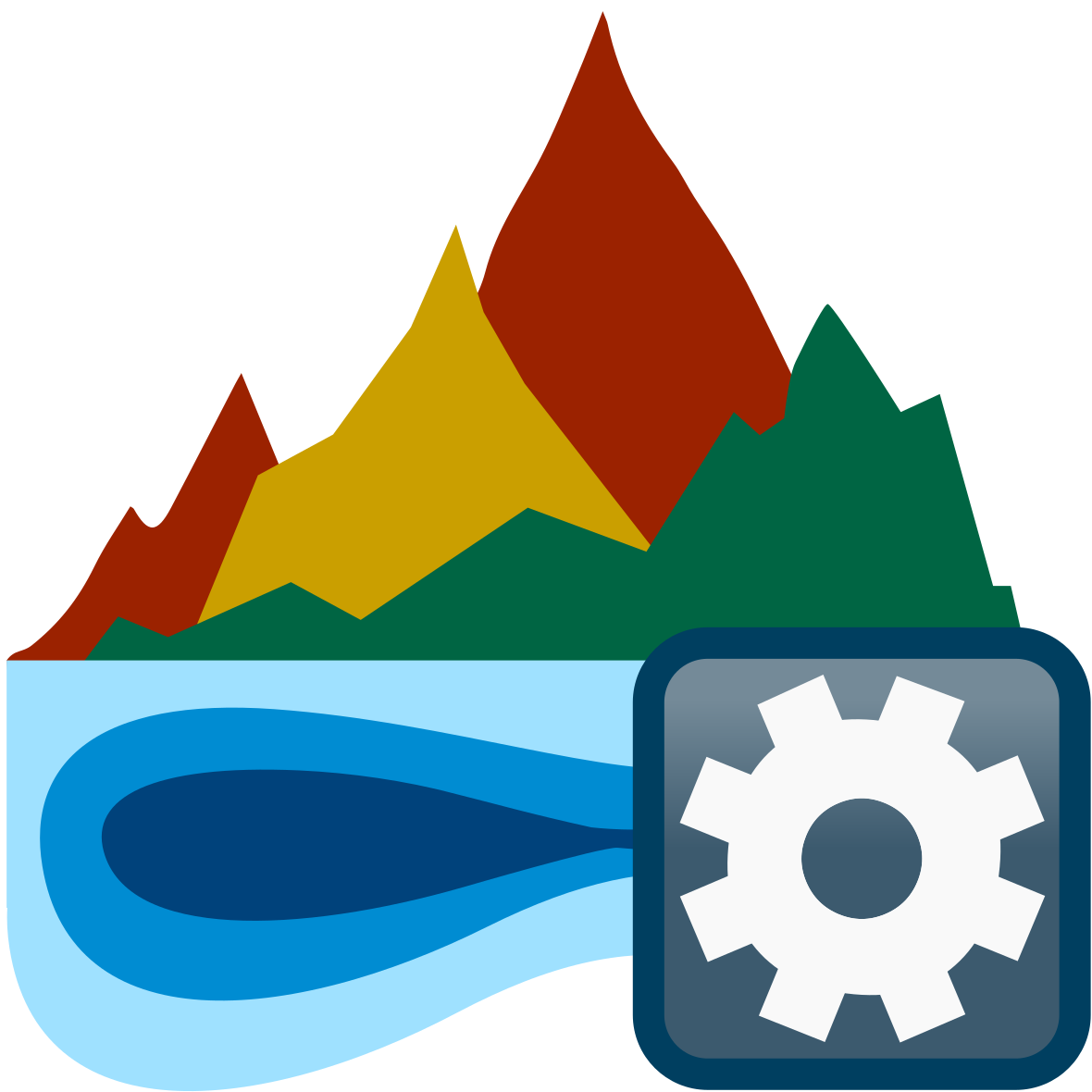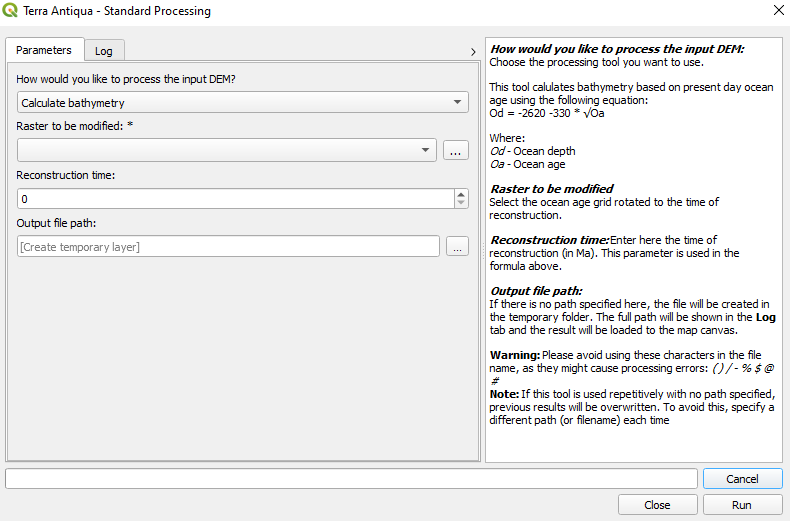Calculate bathyPaleobathymetry can be obtained in two main ways. The simplest one is to use the provided files previously calculated based on geodynamic parameters (Müller et al., 2018; see Primary Tools. In this case you do not need this tool.

This tool will help you if you want to generate your own paleobathymetry based on modern bathymetry extrapolated back in time according to the age of the lithosphere. This will allow keeping many features present today (e.g.: semounts and oceanic plateaus) that would not be in the previously mentioned calculated paleobathymetry (Müller et al., 2018). This tool thus calculates the bathymetry based on present day ocean floor age. For this purpose it uses the following equation:

$Od = 2620 - 330 *Oa$

Where:
Od → Ocean depth
Oa → Ocean age

The Ocean age is calculated based on the time of reconstruction and the ocean age grid. This is a raster file that needs to be entered by the user. It represents the age of the oceanic crust. To calculate the bathymetry, we need the ocean age at the time of reconstruction. Using the Time of the age raster and the Time of reconstruction (in Ma), the ocean age at the time of reconstruction is calculated and used in the formula above.

To better visualize this, here are three possible cases:

Case 1: The raster contains age values for each pixel expressed relative to today but the position of the continents corresponds to 30 Ma

• The time of the age raster is 0 Ma
• The time of reconstruction is 30 Ma
• For the Ocean age used in the formula above, the time of reconstruction (30Ma) is subtracted from the pixel values of the ocean age raster.

Case 2: The raster contains age values for each pixel expressed relative to the time of the reconstruction (30Ma) and the position of the continents corresponds to 30 Ma

• The time of the age raster is 30 Ma
• The time of reconstruction is 30 Ma
• For the Ocean age used in the formula above, the pixel values of the ocean age raster are used directly.

Case 3: The raster contains age values for each pixel expressed relative to a time that doesn’t correspond to the reconstruction (e.g.: 20 Ma) and the position of the continents corresponds to 30 Ma

• The time of the age raster is 20 Ma
• The time of reconstruction is 30 Ma
• For the Ocean age used in the formula above, first the difference between the time of reconstruction and the time of the age raster is calculated. Then the resulting value is subtracted from the pixel values of the ocean age raster. The result is used in the formula above.

## Step by step

1. Click on the icon. The Standard Processing window will pop up.

2. In the field How would you like to process the input DEM, choose Calculate bathymetry.3. In the field Raster to be modified, choose the present-day ocean age grid rotated to the time of reconstruction.

4. In the field Time of the age raster, enter the time the age values of the raster are expressed relative to.

5. In the field Reconstruction time, enter the time of reconstruction in Ma.

6. Choose the Output file path, and click Run.

If you have any comments or discussion, you may add a post here:
Link to general discussions on Terra Antiqua